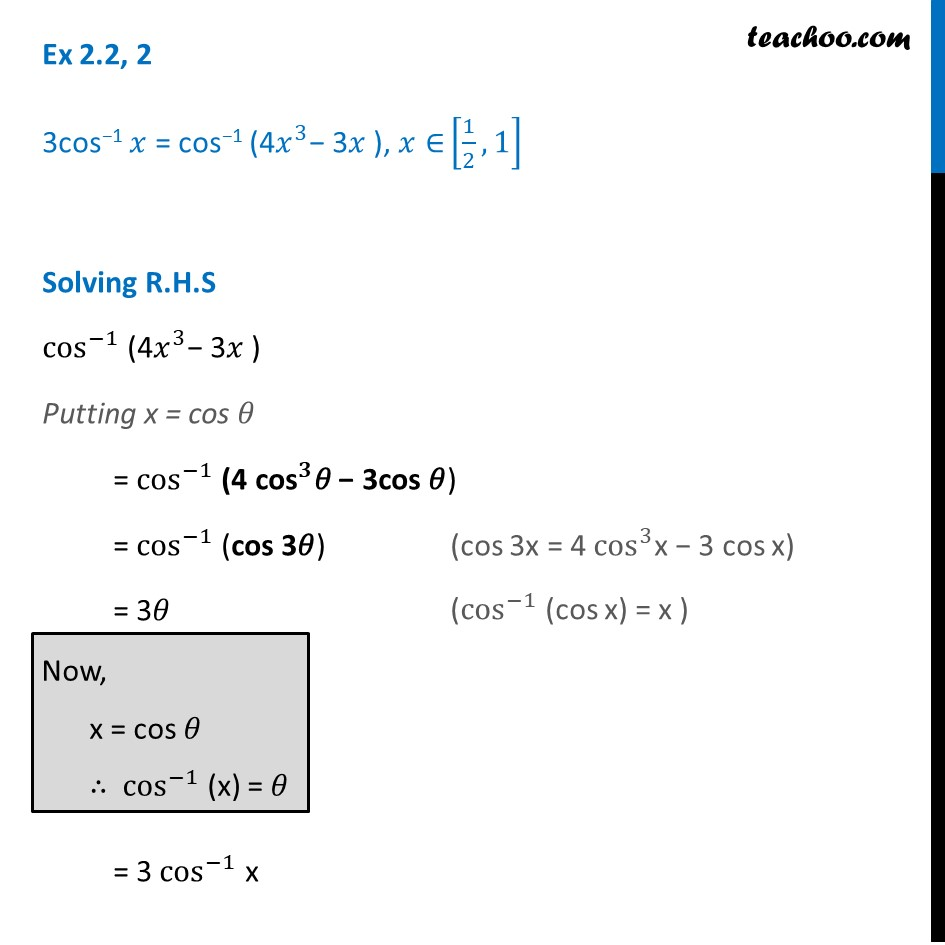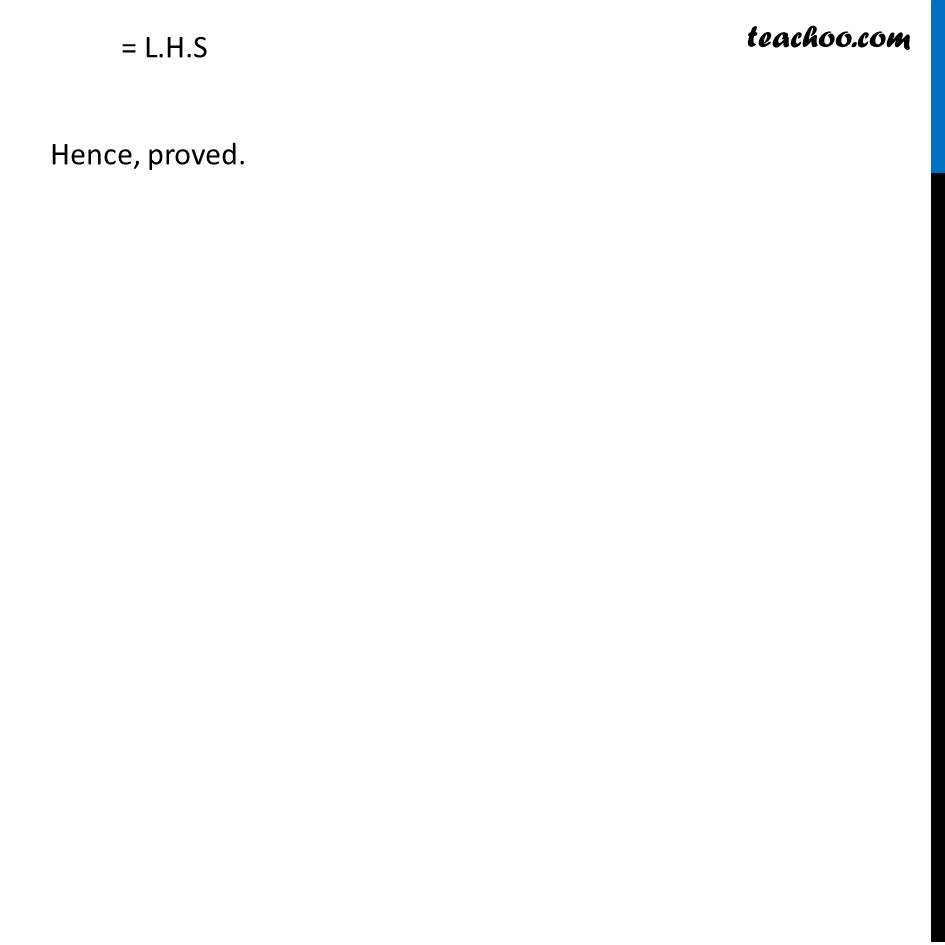Formulae based

Chapter 2 Class 12 Inverse Trigonometric Functions
Concept wiseLearn in your speed, with individual attention - Teachoo Maths 1-on-1 Class

### Transcript

Ex 2.2, 2 3cos−1 𝑥 = cos−1 (4𝑥^3− 3𝑥 ), 𝑥∈ [1/2,1] Solving R.H.S cos^(−1) (4𝑥^3− 3𝑥 ) Putting x = cos 𝜃 = cos^(−1) (4 〖"cos" 〗^𝟑𝜃 − 3cos 𝜃) = cos^(−1) (cos 3𝜃) = 3𝜃 = 3 cos^(−1) x = L.H.S Hence, proved.